# Difference between revisions of "Fixed pulley with vertical loads"

This article discusses a scenario/arrangement whose statics/dynamics/kinematics can be understood using the ideas of classical mechanics.
View other mechanics scenarios

This article describes a special case of a simple pulley system: a fixed pulley with vertical loads connected by a string passing over the pulley, as depicted in the figure.

## Case of a massless pulley and string

In case the pulley and string are massless, the upward tension force exerted by the string on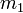$m_1$ equals the upward tension force exerted by the string on$m_2$. If we call this value of tension$T$, then the force diagrams of the two masses are as shown below.

Fill this in later

We have the following cases:

Case Direction of acceleration Magnitude of acceleration Magnitude of tension force$T$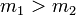$m_1 > m_2$ upward on$m_2$, downward on$m_1$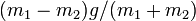$(m_1 - m_2)g/(m_1 + m_2)$$\frac{2m_1m_2g}{m_1 + m_2}$ -- this is$g$ times the harmonic mean of$m_1$ and$m_2$$m_1 < m_2$ downward on$m_2$, upward on$m_1$$(m_2 - m_1)g/(m_1 + m_2)$$\frac{2m_1m_2g}{m_1 + m_2}$ -- this is$g$ times the harmonic mean of$m_1$ and$m_2$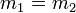$m_1 = m_2$ no direction zero$\frac{2m_1m_2g}{m_1 + m_2}$ -- this is$g$ times the harmonic mean of$m_1$ and$m_2$

Note that the magnitude of the tension force has the same expression in all cases.

KEY FORCE CONCEPT: There is tension in the string in all cases, even when the system is in equilibrium and hence neither mass is accelerating. Tension is what keeps the string taut and in place. Tension goes to zero only when one of the masses goes to zero, which means that the string is no longer taut at that end.

## Case of a massless string and a pulley with moment of inertia

Here's the version in the case that the pulley and the string do not slip against each other. Thus, if$a$ is the acceleration of the string, the angular acceleration of the pulley is$\alpha = a/R$. Note that this requires static friction operating between the string and the pulley. We denote by$I$ the moment of inertia of the pulley and by$R$ the radius of the pulley.

Case Direction of acceleration Magnitude of acceleration in terms of$m_1,m_2,I,R$ Magnitude of acceleration assuming pulley is a solid disk of constant density and mass$M$$m_1 > m_2$ upward on$m_2$, downward on$m_1$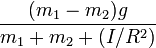$\frac{(m_1 - m_2)g}{m_1 + m_2 + (I/R^2)}$$\frac{(m_1 - m_2)g}{m_1 + m_2 + (M/2)}$$m_2 > m_1$ upward on$m_1$, downward on$m_2$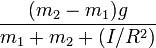$\frac{(m_2 - m_1)g}{m_1 + m_2 + (I/R^2)}$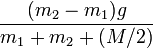$\frac{(m_2 - m_1)g}{m_1 + m_2 + (M/2)}$$m_1 = m_2$ no direction zero (system in equilibrium) zero (system in equilibrium)